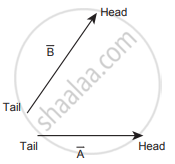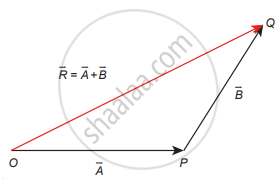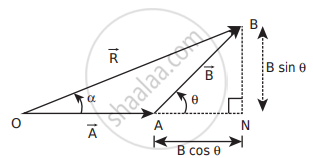Tamil Nadu Board of Secondary EducationHSC Science Class 11th

# Explain in detail the triangle law of addition. - Physics

Explain in detail the triangle law of addition.

#### Solution

Let us consider two vectors vecA and vecB as shown in the figure. To find the resultant of the two vectors we apply the triangular.

present the vectors A and by the two adjacent sides of a triangle taken in the same order. Then the result is given by the third side of the triangle as shown in the figure.To explain further, the head of the first vector vecA is connected to the tail of the second vect vecB Let O he the angle between vecA and vecB. Then vecR is the resultant vector connecting the tail of the first vector vecA to the head of the second vector vecB The magnitude of vecR. (resultant) given geometrically by the length of (OQ) and the direction of the resultant vector is the angle between vecR. and vecA. Thus we write
vecR = vecA + vec(B)  vec(OQ) = vec(OP) + vec(PQ)

1. Magnitude of resultant vector:

The magnitude and angle of the resultant vector are determined by using the triangle law of vectors as follows. From the figure, consider the triangle ABN, which is obtained by extending the side OA to ON. ABN is a right-angled triangle.Resultant vector and its direction by triangle law of addition

From the figure, let R is the magnitude of the resultant of vecA and vecB.
cos θ = "AN"/"B" ∴ AN = B cos θ and sinθ = "BN"/"B" ∴ BN = B sinθ

For ∆ OBN, we have OB2 = ON2 + BN2

⇒ R2 = (A + B cos θ)2 + (B sinθ)2

⇒ R2 = A2 + B2 cos2θ + 2ABcosθ B2 sin2θ

⇒ R2 = A2 + B2(cos2θ + sin2θ) + 2AB cos θ

⇒ R2 = sqrt(A^2 + B^2 + 2ABcostheta)

2. Direction of resultant vectors:

If 0 is the angle between vecA and vecB then,

|vecA + vecB| = sqrt(A^2 + B^2 + 2ABcostheta)

If R makes an angle α with vecA, then in AOBN,

tan α = "BN"/"ON" = "BN"/("OA + AN")

tan α = (Bsintheta)/(A + Bcostheta) ⇒ α = tan^-1((Bsintheta)/(A + Bcostheta))

Concept: Elementary Concept of Vector Algebra
Is there an error in this question or solution?

Share